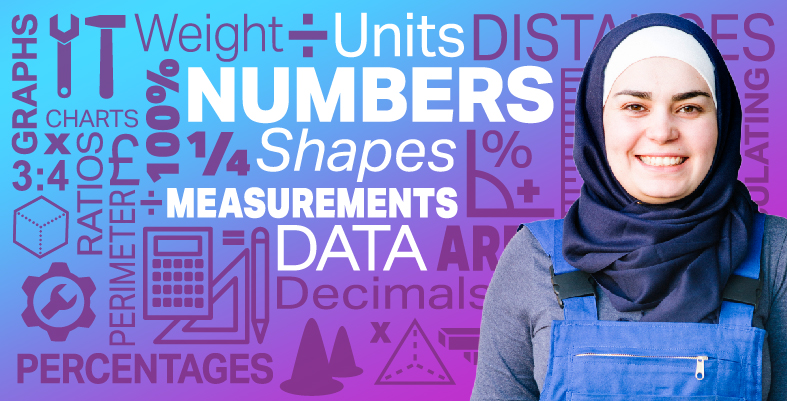Everyday maths for Construction and Engineering 1

Start this free course now. Just create an account and sign in. Enrol and complete the course for a free statement of participation or digital badge if available.

Free course

# 9 Probability

Probability is measuring how likely it is that something will happen. We use probability in different ways in real life:

• Bookmakers use a form of probability to give betting odds on anything.
• Insurance actuaries use probability to decide how much to charge for all the different types of insurance and assurance there is.
• Government departments use probability and statistics to help them govern the country.

(Another word for probability is chance. You might say, ‘What are the chances of this happening?’)

Working through this section will enable you to:

• understand the possibility of different events happening
• show that some events are more likely to occur than others
• understand and use probability scales
• show the probability of events happening using fractions, decimals and percentages.

Probability is measuring how likely it is that something will happen. Look at the word itself: ‘probability’. Can you see it is related to the word ‘probable’?

We know that life is full of choices and chances, or that some things are more likely to happen than others.

For example, you could say, ‘I might cut the grass tomorrow.’ Probability would be used to measure how likely it is that you will cut the grass. There are two options involved here: either you cut the grass or you don’t.

If you knew that it was going to rain tomorrow and you had lots of other things to do (and you hate cutting grass), then the probability of actually cutting the grass would be low or even zero! But on the other hand, if you really intended to cut the grass and the weather forecast was good then the probability of cutting the grass would be high.

We use probability to give us an idea of how likely it is that something will happen. It gives us a measuring system.

• If something is very likely to happen, the probability is high.
• If something is not very likely to happen, the probability is low.

## Example: What are the chances?

What’s the probability of:

• you winning the lottery this week?
• getting wet in the rain?
• summer following spring?

There’s a very low probability that you’ll win the lottery this week, and a high probability of getting wet in the rain and of summer following spring.

Of course, some things have even chances of happening. For example, if you toss a coin, there is an equal probability of it being heads or tails. This could also be called an even chance, or a fifty-fifty chance, of the coin being heads or tails.

How many different things that have different chances of happening can you think of?

Now try the following activity. Remember to check your answers once you have completed the questions.

## Activity 14: Thinking about probability

Take a look at the table, think of some events to add to each of the columns.

Events with a high probability of happening Events with an even chance of happening Events with a low probability of happening
To use this interactive functionality a free OU account is required. Sign in or register.
To use this interactive functionality a free OU account is required. Sign in or register.
To use this interactive functionality a free OU account is required. Sign in or register.
To use this interactive functionality a free OU account is required. Sign in or register.
To use this interactive functionality a free OU account is required. Sign in or register.
To use this interactive functionality a free OU account is required. Sign in or register.
Interactive feature not available in single page view (see it in standard view).

There is no single correct answer to this activity. Have a look at our suggestions below:

Events with a high probability of happening Events with an even chance of happening Events with a low probability of happening
The Moon rising tonightTossing a coin and getting headsWinning the lottery
DeathA baby being born a boyBeing kidnapped by aliens
FSM_SSC_1